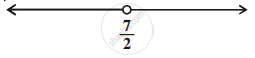# Solution of a linear inequality in variable x is represented on number line given below ______. - Mathematics

MCQ
Fill in the Blanks

Solution of a linear inequality in variable x is represented on number line given below  ______.#### Options

• x ∈ (- oo, 7/2)

• x ∈ (-oo, 7/2]

• x ∈ [7/2, -oo)

• x ∈ (7/2, oo)

#### Solution

Solution of a linear inequality in variable x is represented on number line bbunderline(x ∈ (- oo, 7/2)).

Explanation:

The given graph represents all the values of x less than 7/2 on real number line.

So, x ∈ (- oo, 7/2).

Concept: Algebraic Solutions of Linear Inequalities in One Variable and Their Graphical Representation
Is there an error in this question or solution?

#### APPEARS IN

NCERT Mathematics Exemplar Class 11
Chapter 6 Linear Inequalities
Exercise | Q 29 | Page 110
Share